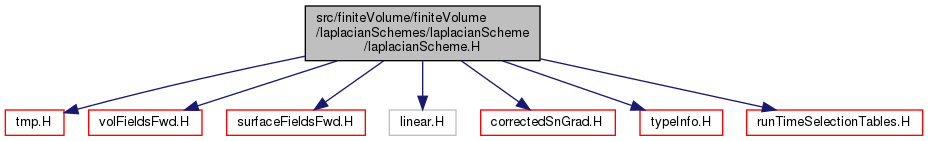The OpenFOAM FoundationlaplacianScheme.H File Reference
Include dependency graph for laplacianScheme.H:This graph shows which files directly or indirectly include this file:Go to the source code of this file.

## Classes

class  fvMatrix< Type >
A special matrix type and solver, designed for finite volume solutions of scalar equations. Face addressing is used to make all matrix assembly and solution loops vectorise. More...

class  laplacianScheme< Type, GType >
Abstract base class for laplacian schemes. More...

## Namespaces

Foam
Namespace for OpenFOAM.

Foam::fv
Namespace for finite-volume.

## Macros

#define makeFvLaplacianTypeScheme(SS, GType, Type)

#define makeFvLaplacianScheme(SS)

## Detailed Description

Original source file laplacianScheme.H

Definition in file laplacianScheme.H.

## ◆ makeFvLaplacianTypeScheme

 #define makeFvLaplacianTypeScheme ( SS, GType, Type )
Value:
typedef Foam::fv::SS<Foam::Type, Foam::GType> SS##Type##GType; \
defineNamedTemplateTypeNameAndDebug(SS##Type##GType, 0); \
\
namespace Foam \
{ \
namespace fv \
{ \
typedef SS<Type, GType> SS##Type##GType; \
\
laplacianScheme<Type, GType>:: \
} \
}
labelList fv(nPoints)
Namespace for OpenFOAM.

Definition at line 215 of file laplacianScheme.H.

## ◆ makeFvLaplacianScheme

 #define makeFvLaplacianScheme ( SS )
Value:
\
makeFvLaplacianTypeScheme(SS, scalar, scalar) \
makeFvLaplacianTypeScheme(SS, symmTensor, scalar) \
makeFvLaplacianTypeScheme(SS, tensor, scalar) \
makeFvLaplacianTypeScheme(SS, scalar, vector) \
makeFvLaplacianTypeScheme(SS, symmTensor, vector) \
makeFvLaplacianTypeScheme(SS, tensor, vector) \
makeFvLaplacianTypeScheme(SS, scalar, sphericalTensor) \
makeFvLaplacianTypeScheme(SS, symmTensor, sphericalTensor) \
makeFvLaplacianTypeScheme(SS, tensor, sphericalTensor) \
makeFvLaplacianTypeScheme(SS, scalar, symmTensor) \
makeFvLaplacianTypeScheme(SS, symmTensor, symmTensor) \
makeFvLaplacianTypeScheme(SS, tensor, symmTensor) \
makeFvLaplacianTypeScheme(SS, scalar, tensor) \
makeFvLaplacianTypeScheme(SS, symmTensor, tensor) \
makeFvLaplacianTypeScheme(SS, tensor, tensor)
Vector< scalar > vector
A scalar version of the templated Vector.
Definition: vector.H:49
SymmTensor< scalar > symmTensor
SymmTensor of scalars.
Definition: symmTensor.H:48
SphericalTensor< scalar > sphericalTensor
SphericalTensor of scalars.
Tensor< scalar > tensor
Tensor of scalars.
Definition: tensor.H:51

Definition at line 232 of file laplacianScheme.H.